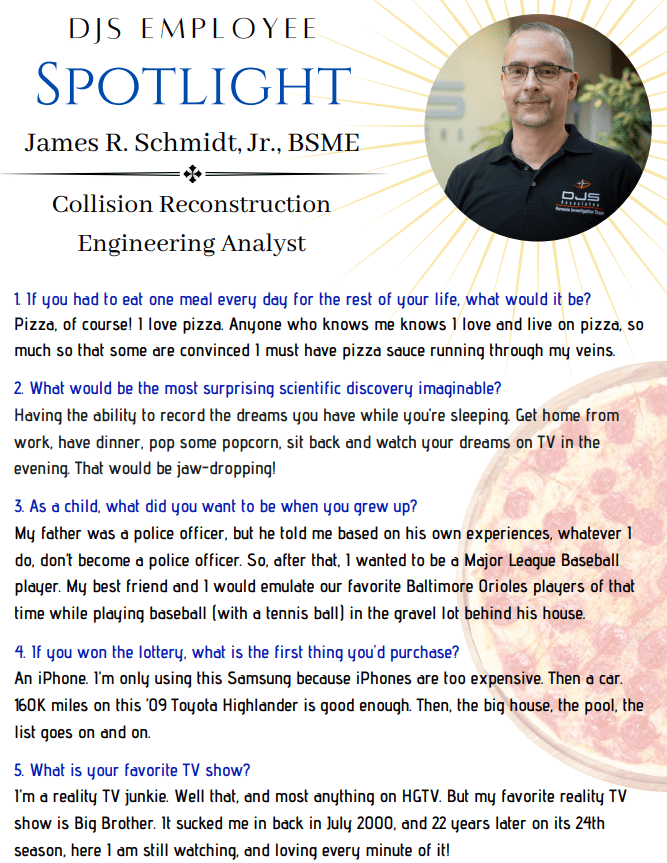,

### Part One: Change in Velocity (delta-V)

:::: James R. Schmidt, Jr., BSME
Sr. Collision Reconstruction Engineer ::::

Change in velocity, also known as delta-V, is a simple concept. It’s just the way it sounds … how much did the velocity (of a vehicle) change during a given event? Let’s take a vehicle travelling at 35 mph. If that vehicle slows to a stop at a red light, it’s change in velocity / delta-V is 35 mph. How long did it take to slow down though? Under normal braking, it would take about 8.0 seconds, which equates to a 0.20 g rate of deceleration (i.e. rate of slowing down). What if that vehicle skids to a stop instead? In that case, it would take about 2.3 seconds, and the rate of deceleration would be 3 ½ times higher at about 0.70 g.

We’re talking about collision reconstruction though, so let’s have this vehicle crash into a fixed object at 35 mph. First, let’s evaluate the scenario in which the vehicle crashes head-on into a utility pole, such that the impact is to the right of center on the front of the vehicle. The 35 mph-worth of forward velocity of this vehicle goes to zero in about 0.11 seconds (give or take). Quite fast, and reflected in the much higher (average) rate of deceleration experienced by the vehicle over such a small increment of time. The g’s are now at 14 ½, which is nearly 21 times faster than skidding to a stop. Next, let’s go one step further. Let’s have the vehicle crash head-on into a brick wall. The forward velocity of the vehicle stops in about 0.08 seconds (plus or minus), which is even quicker than the utility pole impact scenario. Again, as would then be expected, the g-forces are even higher… just about 20 g’s on average, nearly 29 times faster than skidding to a stop, and 100 times faster than normal braking to a stop at a red light. It’s no wonder that severe injuries can result during a collision. (Note: A vehicle has some degree of rebound during a collision which, in the case of crashing into a brick wall for example, results in a delta-V higher than the impact speed alone.)

So, what does all this mean? delta-V is a measure of impact severity, BUT … what type of “impact” are we talking about? You could have the same delta-V for different scenarios. The associated g-forces could be very different, depending on the impact duration. It’s this impact duration, and hence these g-forces calculated by the collision reconstruction engineer, that can then be used by a bio-mechanical expert in the evaluation of injuries sustained by an occupant of a vehicle during a crash. This is delta-V made simple.

### Part Two: Speed from Skid

The fundamental calculation of vehicle speed from a length of skid is rather basic. All you need is the length of skid and the coefficient of friction or drag factor that existed between the vehicle’s tires and the road (or other) surface on which it was traveling. Speed equals the square root of 2 times the acceleration of gravity times the coefficient of friction or drag factor times the skid distance. Sounds a bit complicated? It is really a relatively simple concept and calculation, as you will learn below.

Using the following example, a vehicle skids to a stop just before rear-ending another vehicle at a traffic light. Now, let’s assume the length of skid was 75 feet and it is a typical asphalt roadway that was dry at the time of the collision. A typical coefficient of friction value for this type of roadway would be approximately 0.70. (We say “approximately” because, unless it is measured, there’s always a range associated with such a value.) Inputting that into our “simple” equation above, we calculate the vehicle speed from skid to be 58.1 feet per second. This means that the vehicle was traveling at just over 58 feet every second when it started to skid. What is “feet per second”? Feet per second is just one way of expressing vehicle speed. More commonly, vehicle speed is referred to in miles per hour. So, our vehicle above, traveling at 58.1 feet per second, is traveling at approximately 40 miles per hour (mph). If the posted speed limit on the roadway, as in this example, were 40 mph, then it can be said that the vehicle was traveling around the 40 mph speed limit as it started its skid. If the posted speed limit were more or less than 40 mph, then the corresponding appropriate comparisons can also be made.

There are many other factors taken into consideration when determining speed from skid.

• A typical passenger vehicle (i.e. a car or SUV) takes longer to stop on a wet roadway versus that same vehicle on a dry roadway.
• It would take that vehicle even longer to stop on a snowy or even icy roadway than on a wet roadway.
• A vehicle equipped with an anti-lock braking system (ABS) typically may be able to stop faster (i.e. in a shorter distance) than a vehicle without ABS.
• A passenger vehicle can generally stop much quicker than a commercial vehicle (i.e. a tractor-trailer) on various road surfaces and under various conditions.

So, the type of vehicle (and tires), the type of braking system, the type of roadway, and roadway surface condition (i.e. dry or wet, etc.), all come into play in evaluating vehicle speed from skid. The concept, however, is a basic one.

Categories: Case Studies